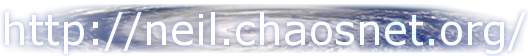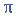We were given a graphing calculator for Math 12. I don't know why, but this is what came of it.

Programming.
Arithmetic Series - slightly useful.
Arithmetic Term - slightly useful.
Cubic Polynomial - forget what it does (read: not useful).
Distance - fairly useful.
Divide Polynomials - slightly useful.
Factor Polynomials - very useful.
Geometric Sequences - slightly useful.
Midpoint - fairly useful.
Percentage Difference - fairly useful.
Recursive Series - slightly useful.
Sequences and Series - slightly useful.
Solve a Triangle - very useful.
Standard Form Quadratics - very useful.
Z3d - useless 3d "graphics" engine.